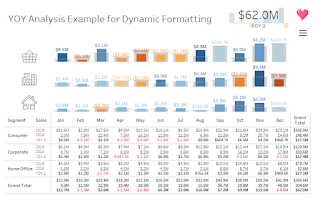### Creating dynamic units of measure formatting is a must-have for any Tableau tips and tricks blog. However, many of these techniques are missing a critical piece.# \$1,234,567,890.00 vs \$1.2B

Being concise is imperative when making fast and informed decisions with data. Using visualizations instead of text is usually the best way to accomplish this. However, users still need to see vital numbers while designers reprove clutter on the dashboard. This is a perfect time to use dynamic units!

# As mentioned above almost every Tableau tips and tricks blog covers dynamic formatting for measure units. But there is one critical piece that many miss.

Many of these dynamic KPIs do not include the absolute value function ABS() in the conditional logic of the calculationNot including this function assumes that the measure will always be positive in the data. This is not necessarily the case - especially in regards to profit or variance calculations.

Let's look at an example. Below is dynamic formatting logic for millions (M) units.

IF SUM([Sales]) >= 1000000 THEN "M" END

Now, suppose it is possible to have negative sales numbers. The logic of this calculation would not recognize the value of -\$1,000,000 to be -\$1M. In fact, the logic above would produce a null. We need to safeguard against this in our calculation by using absolute value.

IF ABS(SUM([Sales])) >= 1000000 THEN "M" END

Perfect! Now we can properly change the value to the unit "M" when it is positive or negative.

Personally, I like to keep my measure value types as numbers and not convert them into strings if possible. This is why I prefer the multiple calculation method with different formatting for KPIs.
Pros:  No rounding or string conversions are necessary.
Cons:  Multiple fields are required for formatting different units of the same value.

Billions:

Millions:

Thousands:

Hundreds or Less:

After the units of measure calculations are created, (i.e., billions, millions, thousands, hundreds or less) we place them all on the same line in the Text label on the Marks Box.

Note: For units of measure that are not listed by default in the Display Units (for example, metric weight in kg), go to the Suffix box in the Custom Number Format and type " kg".

You may download the completed workbook below for several different dynamic units of measure KPIs, including currency or number, time, weight, volume, area, and length:

### Create dynamic measure units for a table.

The above calculations are great for the KPI numbers on our dashboard, but what about when we need to show multiple measures on a text table? Let's say we are doing a year over year (YOY) analysis and we have created different measures for current year, previous year, and the delta. We cannot utilize Measure Values for this scenario and we need to create logic that utilizes rounding, division and string conversion to place the value and the unit of measure together.

Repeat these steps for any other measures needed in the text table.

Note: It may be possible that some of these values are null. This means that we should create a space " " calculation to pad the line in case there is a null.

Next, we place these calculations on in the Text label on the Marks Box.

Uh oh. Now we don't have label headers for our measures! Which number is which on the rows in table below?

No worries. We can create a calculation to act as our measure names. The trick here is to break the line in between the quotation marks as so:

Place this "Label" calculation on Rows and we are good to go.

### Make your dynamic measures more insightful with color.

Now that we have a text table on the dashboard, we definitely need to make it more Tableau-y and add color!

To accomplish this, we do two calculations for each of the "value and unit" calculations that focus on only negative or positive values.

Negative Values:

Positive Values:

We place the negative and positive values for the measure on the same line. I like to use red for the negative values and black for the positive values. And, just like in the step before, we add our space " " calculation to pad the lines in case of null values.

Voila!